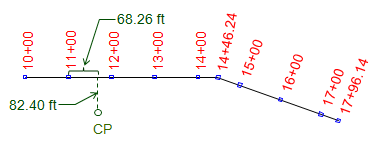### 2. Stationing

A station is both a dimension and a position. As a dimension it has a fixed length with associated unit. As a position it is the cumulative horizontal distance along the alignment from its beginning.

In the English system, a station is defined as the length of a standard surveyor's tape making it 100.00 ft long. A position is expressed as the number of full stations (100 ft intervals) plus a partial station (less than 100 ft) and is written in the full+partial format. Station 8+35.67 is 835.67 feet along the alignment from its origin. Alignments do not usually start at station 0+00 because subsequent redesign can result in negative stations: -1+45.67 looks confusing, doesn't it?

For longer alignments and/or larger stationing values the 1000s format can be used. The number to the left of the + is the number of thousands of feet, to the right is the partial thousand: 2+987.35 corresponds to 2987.35 ft along the alignment.

In the metric system, a station may be 100 m or 1000 m with a corresponding partial station. A point 12,56.02 m along the alignment would be at station 12+26.02 or 1+256.02.

Alignments are traditionally staked at full station (100 ft) intervals, in the English system, and changes in horizontal direction, Figure A-3. In some cases, such as trenching, stakes can be placed at half- or quarter-station intervals (50 ft and 25 ft intervals, respectively).Figure A-3 Stationing

The horizontal distance between two stations along the alignment is their stationing difference.Figure A-4 Distance

In Figure A-4, the distance from sta 12+38.28 to sta 16+56.85 is:The distance can be expressed in feet or stations.

The position of a point off the alignment is expressed by a station and an offset to the right or left (defined looking upstation). The position of the CP in Figure A-5 is 11+68.26 82.40RFigure A-5 Station and Offset

A single point can sometimes have two different stations values. The most common situation is where two different alignments cross, Figure A-6.Figure A-6 Station Equation

Point A is at station 12+83 along one alignment and station 26+45 along a second. A Station Equation can be written for point A: Sta 12+83 = Sta 26+45. This may be brought to the readers' attention on a set of plans to ensure the appropriate station value be used for alignment calulations. Another station equation situation can occur in horizontal curve computations and will be covered in that section.